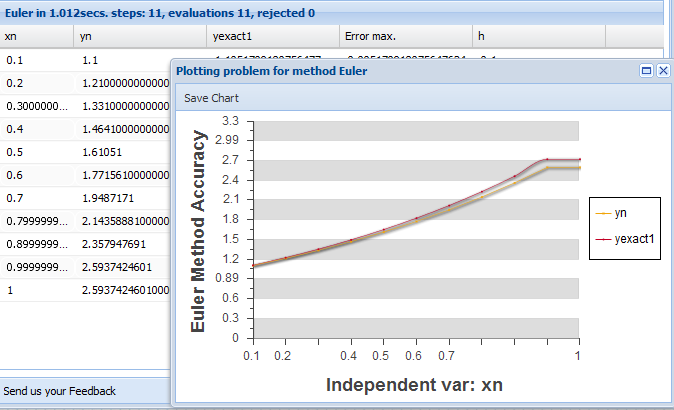# The On-Line Runge-Kutta Calculator

### Basic Concepts and Principles

RungeKutta Calculator is an application developed to calculate numerical solutions in intitial value problems, therefore it search solutions for ODE´s Systems with up to 5 equations.RungeKutta Calculator can solve initial value problems in Ordinary Differential Equations systems up to order 6.

RungeKutta Calculator uses Runge-Kutta, Dormand Prince and Fehlberg pairs embedded methods as explained in this site. The order of these methods is between 1 (Euler method) and 6 (the New65 with FSal property).

### Extension Theory

The window program opens with a default initial value problem

y' = y ,
y(0)= 1

For integration interval: [0, 1]

wich admits the inmediate and obvous solution y=ex

### Inputs

Independent Variable: Enter the starting point t0 and end point tn, wich are the integration interval limits
Dependent Variable: Enter dependent variable initial value x0 (or y0) to start algorithm
function or functions (in the case of a system) that make up the equation, also enter the exact function only if it is known, to estimate the error.
With the Combo ' Select Method': Select integration method to use.

1) Execute Produces the execution of the selected method.
2) Add Dimension Anands a equation to the problem (for system of ODEs).
3) Show Problem Shows our intial value problem with the data introduced.

# Was useful? want add anything?

Post here

### Post from other users

Post here